# Annotations in UWP Charts (SfChart)

10 May 202124 minutes to read

`SfChart` supports `Annotations`, which allows you to mark the specific area of interest in the chart area. You can draw custom shapes, also text and images can be added using `Annotations`.

The following annotations are supported in `SfChart`

You can create an instance for any type of Annotation and add it to `Annotations` collection. Here for instance, the `EllipseAnnotation` is added.

``````<syncfusion:SfChart.Annotations>

<syncfusion:EllipseAnnotation  X1="2.5" Y1="1500"

Text="Ellipse Annotation"

X2="3.6" Y2="1680" >

</syncfusion:EllipseAnnotation>

</syncfusion:SfChart.Annotations>``````
``````EllipseAnnotation annotation=new EllipseAnnotation()
{

X1 = 2.5, Y1 = 1500,

X2 = 3.6, Y2 = 1680,

Text = "Ellipse Annotation"

};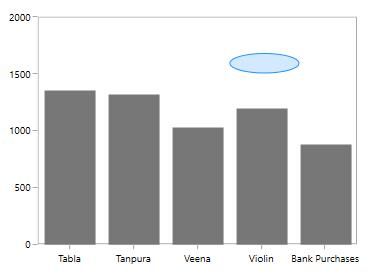## Positioning the Annotation

`Annotations` can be positioned in plot area based on `X1` and `Y1` properties and for image and shape annotations you need to specify `X2` and `Y2` properties. These X and Y values can be specified with axis units or pixel units and this can be identified using `CoordinateUnit` property.

Positioning based on CoordinateUnit as Axis

To position based on axis you need to set the X1 and Y1, X2 and Y2 (if needed) properties based on the primary and secondary axis range values and `CoordinateUnit` as `Axis`.

Positioning based on CoordinateUnit as Pixels

To position based on the pixel values you have to set the `CoordinateUnit` as `Pixels` and the pixel values in X1 and Y1, X2 and Y2 properties in Annotation.

Adding Annotation for MultipleAxes

You can also add annotation for a particular axis when there is multiple axes using `XAxisName` and `YAxisName` properties as in the below code snippet.

``````<Chart:SfChart>

<Chart:SfChart.RowDefinitions>

<Chart:ChartRowDefinition></Chart:ChartRowDefinition>

<Chart:ChartRowDefinition></Chart:ChartRowDefinition>

</Chart:SfChart.RowDefinitions>

<Chart:SfChart.PrimaryAxis>

<Chart:CategoryAxis/>

</Chart:SfChart.PrimaryAxis>

<Chart:SfChart.SecondaryAxis>

<Chart:NumericalAxis

x:Name="FirstYAxis"

Chart:ChartBase.Row="0"/>

</Chart:SfChart.SecondaryAxis>

<Chart:SfChart.Annotations>

<Chart:HorizontalLineAnnotation X1="-0.5" X2="3.5"

Stroke="DarkGray"

StrokeThickness="2"

LineCap="Arrow"

CanDrag="True" CanResize="True"

YAxisName="FirstYAxis"

Y1="500">

</Chart:HorizontalLineAnnotation>

<Chart:RectangleAnnotation  X1="0.6" CanDrag="True" CanResize="True"

X2="2.2" Y2="1500" Y1="1800"

Stroke="DarkGray"

Fill="LightGray"

Opacity="0.5"

YAxisName="SecondYAxis">

</Chart:RectangleAnnotation>

</Chart:SfChart.Annotations>

<Chart:ColumnSeries  Label="2011" Interior="#777777"

ItemsSource="{Binding Demands}"

XBindingPath="Demand"

YBindingPath="Year2011"/>

<Chart:ScatterSeries  Interior="#777777" ItemsSource="{Binding Demands}"

XBindingPath="Demand"  YBindingPath="Year2010">

<Chart:ScatterSeries.YAxis>

<Chart:NumericalAxis x:Name="SecondYAxis"

LabelTemplate="{StaticResource label}" TickLineSize="5"

MajorTickLineStyle="{StaticResource tick}" Minimum="0" Maximum="2000" Interval="500"

AxisLineStyle="{StaticResource axis}" ShowGridLines="False"

Chart:ChartBase.Row="1"></Chart:NumericalAxis>

</Chart:ScatterSeries.YAxis>

</Chart:ScatterSeries>``````
``````SfChart chart = new SfChart();

chart.PrimaryAxis = new CategoryAxis();

chart.SecondaryAxis = new NumericalAxis();

ChartBase.SetRow(chart.SecondaryAxis, 0);

HorizontalLineAnnotation annotation = new HorizontalLineAnnotation()
{

X1 = -0.5, Y1 = 500,

X2 = 3.5,

Stroke = new SolidColorBrush(Colors.DarkGray),

StrokeThickness = 2,

LineCap = LineCap.Arrow,

CanDrag = true,

CanResize = true,

YAxisName = "FirstYAxis"

};

RectangleAnnotation annotation1 = new RectangleAnnotation()
{

X1 = 0.6,Y1 = 1500,

X2 = 2.2,Y2=1800,

Stroke = new SolidColorBrush(Colors.DarkGray),

Fill = new SolidColorBrush(Colors.LightGray),

StrokeThickness = 2,

Opacity = 0.5,

CanDrag = true,

CanResize = true,

YAxisName = "SecondYAxis"

};

ColumnSeries columnSeries = new ColumnSeries()
{

ItemsSource = new ViewModel().Demands,

XBindingPath = "Demand",

YBindingPath = "Year2011",

Interior = new SolidColorBrush(Color.FromRgb(0x77, 0x77, 0x77))

};

ScatterSeries scatterSeries = new ScatterSeries()
{

ItemsSource = new ViewModel().Demands,

XBindingPath = "Demand",

YBindingPath = "Year2010",

Interior = new SolidColorBrush(Color.FromRgb(0x77, 0x77, 0x77))

};

NumericalAxis axis = new NumericalAxis()
{

Minimum = 0,

Maximum = 2000,

Interval = 500,

ShowGridLines = false,

TickLineSize = 5,

LabelTemplate = chart.Resources["labelTemplate"] as DataTemplate,

MajorTickLineStyle = chart.Resources["lineStyle1"] as Style,

AxisLineStyle = chart.Resources["lineStyle2"] as Style

};

ChartBase.SetRow(axis, 1);

scatterSeries.YAxis = axis;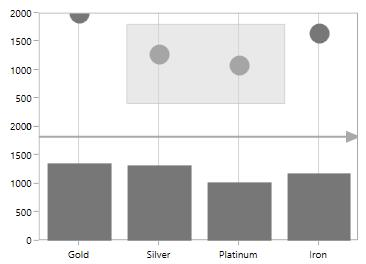## Aligning the Annotation

You can align the annotation horizontally and vertically from its actual plotting position using the `HorizontalAlignment` and `VerticalAlignment` properties as in the following code sample.

• XAML
• ``````<syncfusion:SfChart.Annotations>

<syncfusion:RectangleAnnotation  X1="0.6"

HorizontalAlignment="Left"

VerticalAlignment="Bottom"

X2="2.2"

Y2="1500"

Y1="1800"

Stroke="DarkGray"

Fill="LightGray"

Opacity="0.5">

</syncfusion:RectangleAnnotation>

</syncfusion:SfChart.Annotations>``````

In the following image, you can see the rectangle annotation aligned to the bottom left from its actual position in the chart area.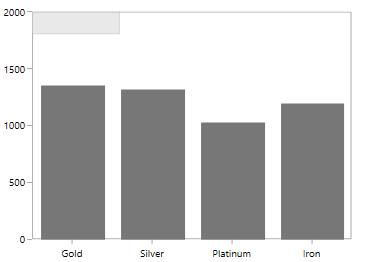## Text Annotation

`TextAnnotations` are used to add simple text in specific points over the chart area.

### Rotate the Text Annotation

The `Angle` property is used to get or set the angle for rotating the Annotation. The following code example demonstrate the rotation feature for `TextAnnotation`.

• XAML
• ``````<syncfusion:SfChart.Annotations>

<syncfusion:TextAnnotation  CoordinateUnit="Axis"

Text="Annotation"

HorizontalAlignment="Stretch"

VerticalAlignment="Stretch"

FontWeight="Bold"

Foreground="Black"

Angle="90"

X1="3.5" Y1="500" >

</syncfusion:TextAnnotation>

</syncfusion:SfChart.Annotations>``````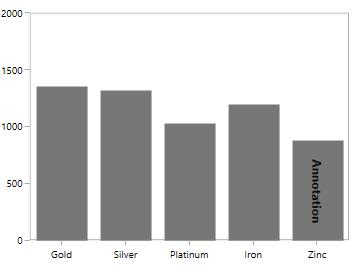### Editing Text Annotation

`SfChart` provides you with an editing option for the text in any annotations. When text annotation is enabled editing, if we click the text annotation it switches to edit mode which provide easy way of customizing the text at run time. You can enable the editing mode in TextAnnotation using `EnableEditing` property.

The following code example and screenshot demonstrate `TextAnnotation` while editing the text.

• XAML
• ``````<syncfusion:SfChart.Annotations>

<syncfusion:TextAnnotation  CoordinateUnit="Axis"

Text="Annotation"

EnableEditing="True"

HorizontalAlignment="Stretch"

VerticalAlignment="Stretch"

FontWeight="Bold"

Foreground="Black"

Angle="90"

X1="3.5" Y1="500" >

</syncfusion:TextAnnotation>

</syncfusion:SfChart.Annotations>``````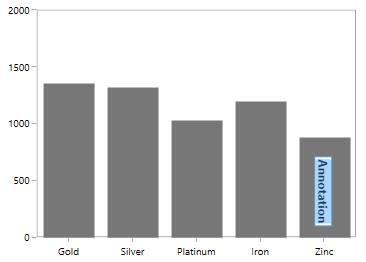## Shape Annotation

`ShapeAnnotation` allows you to add annotations in the form of shapes such as rectangle, ellipse,horizontal line and vertical line at the specific area of interest, in the chart area.

The following API’s are commonly used in all ShapeAnnotation:

### Customization options for LineAnnotation

GrabExtent

`GrabExtent` property of `LineAnnotation` allows user to extent the hit visible area, while performing dragging and resizing. We need to set the desired pixel value for `GrabExtent` property and it can be set as shown in the below code example:

``````<syncfusion:SfChart.Annotations>

<syncfusion:LineAnnotation X1="0" X2="3" Y1="1500" Y2="1500"
GrabExtent="10"
Stroke="DarkGray" CanDrag="True"
CanResize="True"/>

</syncfusion:SfChart.Annotations>``````
``````LineAnnotation annotation = new LineAnnotation()
{
X1 = 0,
X2 = 3,
Y1 = 1500,
Y2 = 1500,
CanDrag = true,
Stroke = new SolidColorBrush(Colors.DarkGray),
CanResize = true,
GrabExtent = 10
};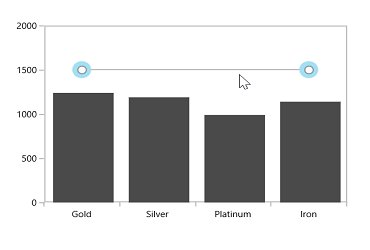Toggling LineAnnotation Visibility

`LineAnnotation` by default will be visible. You can also collapse the visibility of the line annotation using `ShowLine` property.

• XAML
• ``````<syncfusion:SfChart.Annotations>

<syncfusion:HorizontalLineAnnotation X1="-0.5" X2="3.5"

Stroke="DarkGray"

StrokeThickness="1"

ShowLine="False"

Fill="LightGray"

Y1="1500" >

</syncfusion:HorizontalLineAnnotation>

<syncfusion:VerticalLineAnnotation  Stroke="DarkGray" StrokeThickness="1"

X1="1.5" >

</syncfusion:VerticalLineAnnotation>

</syncfusion:SfChart.Annotations>``````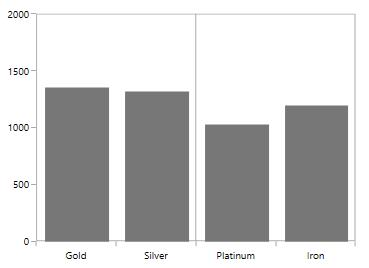Displaying Axis Labels for LineAnnotation

`VerticalLineAnnotation` and `HorizontalLineAnnotation` also displays the axis labels in which the line is placed. This feature can be enabled by setting `ShowAxisLabel` property to true as in the below code snippet.

• XAML
• ``````<syncfusion:SfChart.Annotations>

<syncfusion:HorizontalLineAnnotation X1="-0.5" X2="3.5"

Stroke="DarkGray"

StrokeThickness="1"

ShowAxisLabel="True"

Fill="LightGray"

Y1="1500" >

</syncfusion:HorizontalLineAnnotation>

<syncfusion:VerticalLineAnnotation  Stroke="DarkGray"

StrokeThickness="1"

ShowAxisLabel="True"

X1="1.5" >

</syncfusion:VerticalLineAnnotation>

</syncfusion:SfChart.Annotations>``````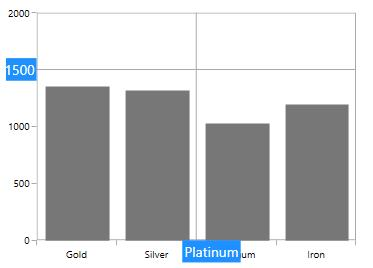AxisLabelTemplate

You can also customize the default appearance of the axis label using `AxisLabelTemplate` property as in the below code snippet.

• XAML
• ``````<syncfusion:SfChart.Annotations>

<syncfusion:HorizontalLineAnnotation X1="-0.5" X2="3.5"

Stroke="DarkGray"

StrokeThickness="1"

ShowAxisLabel="True"

Y1="1500" >

<syncfusion:HorizontalLineAnnotation.AxisLabelTemplate>

<DataTemplate>

<Border BorderBrush="DarkGray"

Background="LightGray">

<TextBlock Foreground="Black"

FontSize="11"

Text="{Binding}">

</TextBlock>

</Border>

</DataTemplate>

</syncfusion:HorizontalLineAnnotation.AxisLabelTemplate>

</syncfusion:HorizontalLineAnnotation>

</syncfusion:SfChart.Annotations>``````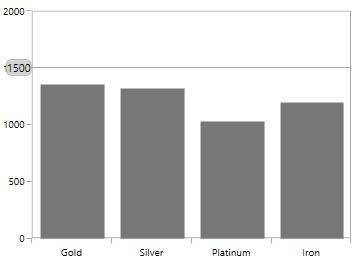Adding Arrow to LineAnnotation

To display single headed arrow you can modify the line annotation by setting `LineCap` property to `Arrow`. By default the `LineCap` property value in none.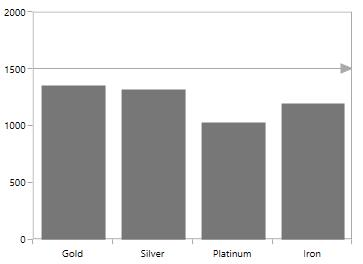### Adding Text in ShapeAnnotation

For all the annotations like Text, Shape, Image and Line, you can display the text for the annotation using Text property as in the below code snippet.

• XAML
• ``````<syncfusion:SfChart.Annotations>

<syncfusion:EllipseAnnotation  X1="2.5" Y1="1500"

Fill="LightBlue" Text="Annotation"

X2="3.6" Y2="1680" >

</syncfusion:EllipseAnnotation>

</syncfusion:SfChart.Annotations>``````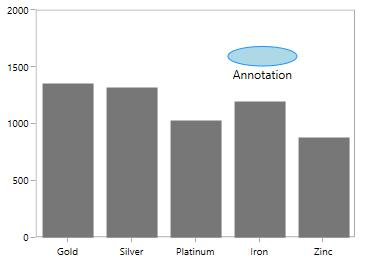### Aligning Text in ShapeAnnotation

The text alignment can be changed using `HorizontalTextAlignment` and `VerticalTextAlignment` properties.

EllipseAnnotation

• XAML
• ``````<syncfusion:SfChart.Annotations>

<syncfusion:EllipseAnnotation  X1="2.5" Y1="1500"

HorizontalTextAlignment="Center"

VerticalTextAlignment="Center"

Fill="LightBlue" Text="Annotation"

X2="3.6" Y2="1680" >

</syncfusion:EllipseAnnotation>

</syncfusion:SfChart.Annotations>``````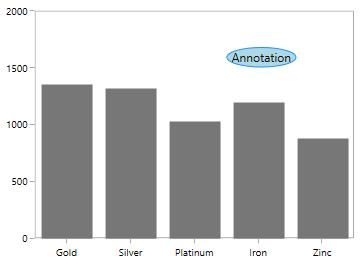Line Annotation

• XAML
• ``````<syncfusion:SfChart.Annotations>

<syncfusion:HorizontalLineAnnotation Text="Line Annotation"

X1="2" X2="3.5"

Stroke="Black"

StrokeThickness="1"

HorizontalTextAlignment="Center"

VerticalTextAlignment="Bottom"

Y1="1500" >

</syncfusion:HorizontalLineAnnotation>

</syncfusion:SfChart.Annotations>``````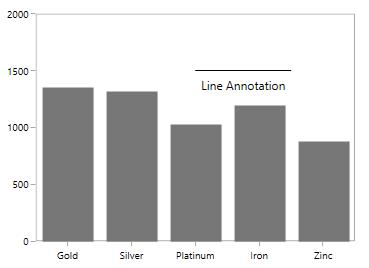Image Annotation

• XAML
• ``````<syncfusion:SfChart.Annotations>

<syncfusion:ImageAnnotation  Text="Annotation"

HorizontalTextAlignment="Center"

VerticalTextAlignment="Top"

X1="2.5" Y1="1200" X2="3.6" Y2="1700" >

</syncfusion:ImageAnnotation>

</syncfusion:SfChart.Annotations>``````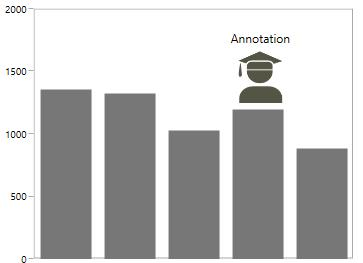NOTE

`HorizontalTextAlignment` and `VerticalTextAlignment` properties are not applicable for `TextAnnotation`.

### Customization of ShapeAnnotation

SfChart allows customization of shape annotation using the following properties.

• XAML
• ``````<syncfusion:SfChart.Annotations>

<syncfusion:HorizontalLineAnnotation X1="-0.5" X2="3.5"

Stroke="DarkGray"

StrokeThickness="3"

HorizontalTextAlignment="Center"

VerticalTextAlignment="Bottom"

Fill="LightGray"

StrokeDashArray="1,1"

StrokeStartLineCap="Square"

StrokeEndLineCap="Square"

StrokeDashCap="Round"

StrokeDashOffset="1.5"

Y1="1500" >

</syncfusion:HorizontalLineAnnotation>

</syncfusion:SfChart.Annotations>``````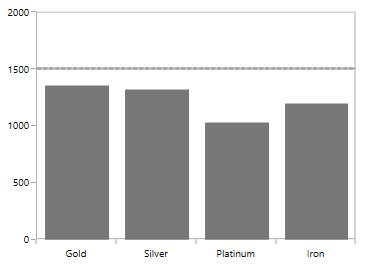## Image Annotation

`SfChart` provides support to add images as Annotation over the chart area, using the class ImageAnnotation.

The following API’s are used in ImageAnnotation

• XAML
• ``````<syncfusion:SfChart.Annotations>

<syncfusion:ImageAnnotation  CoordinateUnit="Axis"

ImageSource="apple.png"

X1="3" Y1="1200" X2="4" Y2="1450" />

<syncfusion:ImageAnnotation  CoordinateUnit="Axis" ImageSource="Fruit-Banana-01.png"

X1="2" Y1="1000" X2="3" Y2="1400"/>

<syncfusion:ImageAnnotation  CoordinateUnit="Axis" ImageSource="Fruit-Grapes.png"

HorizontalAlignment="Center"

VerticalAlignment="Center"

X1="1" Y1="1350" X2="2" Y2="1750" />

<syncfusion:ImageAnnotation  CoordinateUnit="Axis" ImageSource="Fruit-Strawberry.png"

HorizontalAlignment="Center"

VerticalAlignment="Center"

X1="0" Y1="1350" X2="1" Y2="1600" >

</syncfusion:ImageAnnotation>

</syncfusion:SfChart.Annotations>``````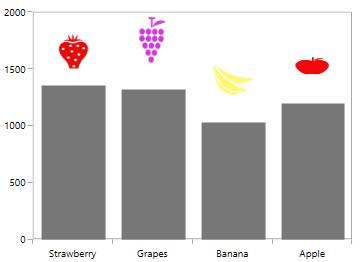Rotating the image in ImageAnnotation

• XAML
• ``````<syncfusion:SfChart.Annotations>

<syncfusion:ImageAnnotation  Angle="-90" CoordinateUnit="Axis" ImageSource="apple.png"

HorizontalAlignment="Center"

VerticalAlignment="Top"

X1="3" Y1="1300" X2="3.5" Y2="1450" />

<syncfusion:ImageAnnotation  Angle="-90" CoordinateUnit="Axis" ImageSource="Fruit-Banana-01.png"

HorizontalAlignment="Center"

VerticalAlignment="Center"

X1="2" Y1="1000" X2="2.5" Y2="1400"/>

<syncfusion:ImageAnnotation   Angle="-90" CoordinateUnit="Axis" ImageSource="Fruit-Grapes.png"

HorizontalAlignment="Center"

VerticalAlignment="Center"

X1="1" Y1="1350" X2="1.5" Y2="1750" />

<syncfusion:ImageAnnotation   Angle="-90" CoordinateUnit="Axis" ImageSource="Fruit-Strawberry.png"

HorizontalAlignment="Center"

VerticalAlignment="Center"

X1="0" Y1="1350" X2="0.5" Y2="1650" >

</syncfusion:ImageAnnotation>

</syncfusion:SfChart.Annotations>``````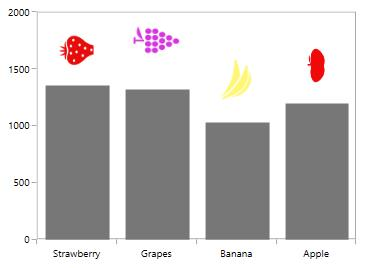## Interactivity

### ToolTip

`SfChart` provides support to view the tooltip when mouse hovered on the annotation. To view to tooltip you have to enable the `ShowToolTip` property. By default for tooltip there is no content, you have to set the content for the tooltip in `ToolTipContent` property.

The following code example demonstrates the default tooltip.

• XAML
• ``````<chart:SfChart.Annotations>

<syncfusion:EllipseAnnotation  X1="2.5" Y1="1500"

Stroke="DarkGray" ToolTipContent="Annotation"

Fill="LightGray" ShowToolTip="True"

X2="3.6" Y2="1680">

</syncfusion:EllipseAnnotation>

</syncfusion:SfChart.Annotations>``````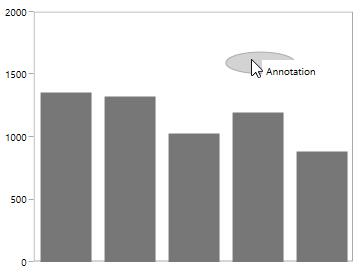Position the Tooltip

Tooltip can be placed top, bottom, left or right side of the cursor using `ToolTipPlacement` property. The tooltip by default will be placed in Right. The following code example demonstrates the tooltip placed at the bottom.

• XAML
• ``````<syncfusion:SfChart.Annotations>

<syncfusion:EllipseAnnotation  X1="2.5" Y1="1500"

Stroke="DarkGray" ToolTipContent="Annotation"

ToolTipPlacement="Bottom"

Fill="LightGray" ShowToolTip="True"

X2="3.6" Y2="1680" >

</syncfusion:EllipseAnnotation>

</syncfusion:SfChart.Annotations>``````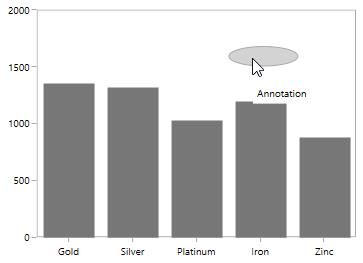ToolTipTemplate

The default appearance of the Tooltip can be changed using `TooltipTemplate` property as in the below code snippet.

• XAML
• ``````<syncfusion:SfChart.Annotations>

<syncfusion:EllipseAnnotation  X1="2.5" Y1="1500"

Stroke="DarkGray"

Fill="LightGray" ShowToolTip="True"

X2="3.6" Y2="1680">

<syncfusion:EllipseAnnotation.ToolTipTemplate>

<DataTemplate>

BorderThickness="1">

<TextBlock FontSize="11" Text="Annotation"

Foreground="Black">

</TextBlock>

</Border>

</DataTemplate>

</syncfusion:EllipseAnnotation.ToolTipTemplate>

</syncfusion:EllipseAnnotation>

</syncfusion:SfChart.Annotations>``````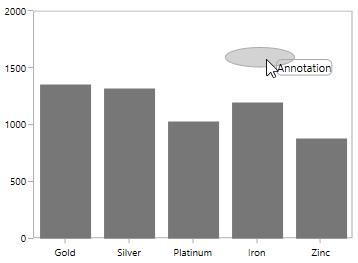### Drag and Resize the Annotation

`SfChart` provides dragging and resizing support for `ShapeAnnotations`.

The following API’s are used for dragging and resizing the annotation

Dragging the Annotation

The following code example demonstrates the dragging the rectangle annotation.

• XAML
• ``````<syncfusion:SfChart.Annotations>

<syncfusion:RectangleAnnotation  X1="0.6" CanDrag="True"

X2="2.2" Y2="1500"

Y1="1800"

Stroke="DarkGray"

Fill="LightGray"

Opacity="0.5">

</syncfusion:RectangleAnnotation>

</syncfusion:SfChart.Annotations>``````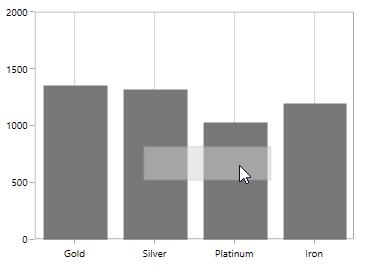You can set the `DraggingMode` as Horizontal which will limit the annotation to be dragged only by horizontally as in the below code snippet.

• XAML
• ``````<syncfusion:SfChart.Annotations>

<syncfusion:RectangleAnnotation  X1="0.6" CanDrag="True"

X2="2.2" Y2="1500"

Y1="1800"

DraggingMode="Horizontal"

Stroke="DarkGray"

Fill="LightGray"

Opacity="0.5">

</syncfusion:RectangleAnnotation>

</syncfusion:SfChart.Annotations>``````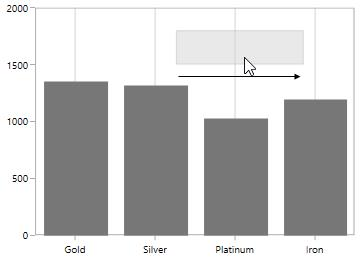Resizing the Annotation

You can resize the annotation by enabling `CanResize` property to True as in the below code snippet.

• XAML
• ``````<syncfusion:SfChart.Annotations>

<syncfusion:RectangleAnnotation  X1="0.6"

CanResize="True"

X2="2.2"

Y2="1500"

Y1="1800"

Stroke="DarkGray"

Fill="LightGray"

Opacity="0.5">

</syncfusion:RectangleAnnotation>

</syncfusion:SfChart.Annotations>``````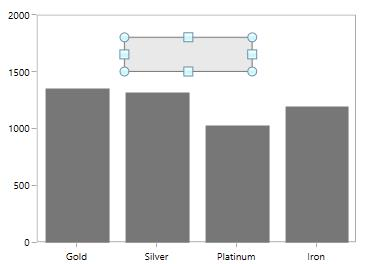`ResizingMode` allows you to limit the resizing to a particular direction may be horizontal, vertical or both direction. The following code example demonstrates the resizing of annotation along vertical direction.

• XAML
• ``````<syncfusion:SfChart.Annotations>

<syncfusion:RectangleAnnotation  X1="0.6"

CanResize="True"

X2="2.2"

Y2="1500"

ResizingMode="Vertical"

Y1="1800"

Stroke="DarkGray"

Fill="LightGray"

Opacity="0.5">

</syncfusion:RectangleAnnotation>

</syncfusion:SfChart.Annotations>``````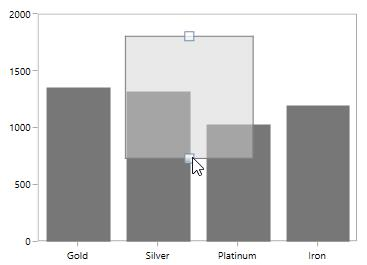## Annotation Clipping

`SfChart` allows you to clip the annotation if the annotation crosses the boundary by setting `EnableClipping` property to True as in the below code snippet.

• XAML
• ``````<syncfusion:SfChart.Annotations>

<syncfusion:ImageAnnotation  HorizontalTextAlignment="Center"

VerticalTextAlignment="Top"

EnableClipping="True"

X1="4" Y1="1200" X2="7" Y2="1700" >

</syncfusion:ImageAnnotation>

</syncfusion:SfChart.Annotations>``````

The following screenshot explains that even when x value is provided out of bounds the image annotation is placed inside the chart area.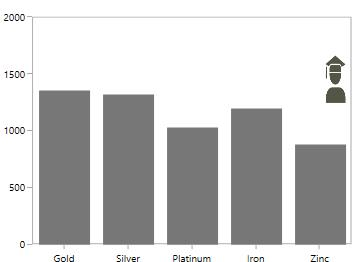## Customizing Text in Annotation

The text in annotation can be customized using the following API’s

The following code example demonstrates the customization of annotation text.

• XAML
• ``````<syncfusion:SfChart.Annotations>

<syncfusion:EllipseAnnotation  X1="2.5" Y1="1500"

Stroke="DarkGray"

Text="Annotation"

FontSize="12" Foreground="DarkGray"

FontStyle="Italic" FontWeight="Bold"

Fill="LightGray" ShowToolTip="True"

X2="3.6" Y2="1680">

</syncfusion:EllipseAnnotation>

</syncfusion:SfChart.Annotations>``````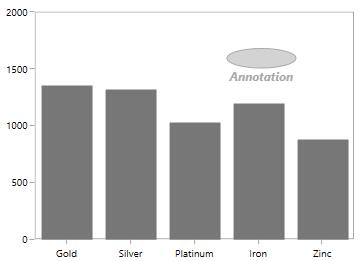Text can also be customized by setting `ContentTemplate` property as in the below code snippet.

• XAML
• ``````<syncfusion:SfChart.Annotations>

<syncfusion:TextAnnotation  CoordinateUnit="Pixel"

X1="266" Y1="4" >

<syncfusion:TextAnnotation.ContentTemplate>

<DataTemplate>

<Border Height="20" Width="80" BorderBrush="Black" CornerRadius="2" Background="LightGray" >

<TextBlock HorizontalAlignment="Center" VerticalAlignment="Center"

FontFamily="Calibri (Body)"

FontWeight="SemiBold" Foreground="Black" FontSize="12"

Text="Gold Demand" FontStyle="Italic"></TextBlock>

</Border>

</DataTemplate>

</syncfusion:TextAnnotation.ContentTemplate>

</syncfusion:TextAnnotation>

</syncfusion:SfChart.Annotations>``````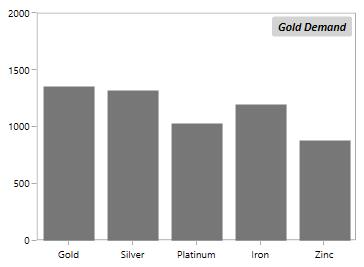## Events

`SfChart` provides the following events in `Annotation`.